# Black building

Keith built building with a rectangular shape 6.5 m × 3.9 m. Calculate how much percent exceeded the limit 25 m2 for small building. Building not built in accordance with the law is called "black building". Calculate the angle that the walls were clenching each other to meet limit footprint limit 25 m2 with given lengths of the walls, if it stands angular.

p =  1.4 %
α =  80.5 °

### Step-by-step explanation:

$p=100\frac{6.5\cdot 3.9-25}{25}=1.4\mathrm{%}$
$\alpha =\mathrm{arcsin}\left(\frac{25}{6.5\cdot 3.9}\right)=80.5\text{°}$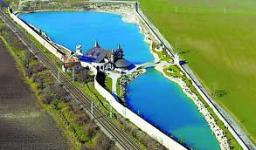Did you find an error or inaccuracy? Feel free to write us. Thank you!Tips to related online calculators
Do you want to convert length units?
Most natural application of trigonometry and trigonometric functions is a calculation of the triangles. Common and less common calculations of different types of triangles offers our triangle calculator. Word trigonometry comes from Greek and literally means triangle calculation.

#### You need to know the following knowledge to solve this word math problem:

We encourage you to watch this tutorial video on this math problem:

## Related math problems and questions:

• Rhomboid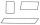Calculate the circumference and area of the rhomboid with sides 20 and 14, with their angle 50°.
• Built-up areaJohn build up area 5 x 7 = 35 m2 with building with a wall thickness 30 cm. How many centimeters would have to subtract from thickness of the walls that built-up area fell by 9%?
• Rhomboid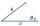The rhomboid sides' dimensions are a= 5cm, b = 6 cm, and the angle's size at vertex A is 60°. What is the length of the side AC?
• Tiles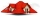The pupils of the building school have to calculate how many roof tiles they will need to cover the roof of the house in the shape of two rectangles measuring 10 x 7.2 m. One roof tile covers a rectangular area of 22 cmx32 cm. How many tiles will they nee
• Trapezoid MOThe rectangular trapezoid ABCD with the right angle at point B, |AC| = 12, |CD| = 8, diagonals are perpendicular to each other. Calculate the perimeter and area of ​​the trapezoid.
• Diagonal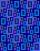The diagonal of the rectangle has a length of 41.4 cm. The angle between the diagonal and longer side of the rectangle is 26°. Calculate the area of the rectangle.
• Office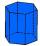Office building was built in the shape of a regular hexagon inscribed in a circle with a radius of 12 m. The height of the walls is 7m. How much CZK cost plastering the walls of the building, if per 1 m square cost CZK 400?
• BuildingThe building I focused at an angle 30°. When I moved 5 m building I focused at an angle 45°. What is the height of the building?What is the volume of a quadrilateral oblique prism with base edges of length a = 1m, b = 1.1m, c = 1.2m, d = 0.7m, if a side edge of length h = 3.9m has a deviation from the base of 20° 35' and the edges a, b form an angle of 50.5°.
• SSA and geometryThe distance between the points P and Q was 356 m measured in the terrain. The PQ line can be seen from the viewer at a viewing angle of 107° 22 '. The observer's distance from P is 271 m. Determine the viewing angle of P and observer.
• Percent changeIf the length of a rectangle is increased by 25% and the width is decreased by 10%, the area of the rectangle is larger than the area of the original rectangle by what percent?
• Jewel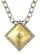Rhombus-shaped jewel has an area of 93 mm2 and the edge in long 13.2 mm. Calculate the size of the rhombus acute angle.
• ParallelogramCalculate area of the parallelogram ABCD as shown if |AB| = 19 cm, |BC| = 18 cm and angle BAD = 90°
• RiverFrom the observatory 11 m high and 24 m from the riverbank, river width appears in the visual angle φ = 13°. Calculate the width of the river.
• RectangleCalculate the length of the side GN and diagonal QN of rectangle QGNH when given: |HN| = 25 cm and angle ∠ QGH = 28 degrees.
• Children playgroundThe playground has a trapezoid shape, and the parallel sides have a length of 36 m and 21 m. The remaining two sides are 14 m long and 16 m long. Find the size of the inner trapezoid angles.What is the slope of a ladder 6.2 m long and 5.12 m in height.